Miscellaneous

Chapter 11 Class 12 Three Dimensional Geometry
Serial order wise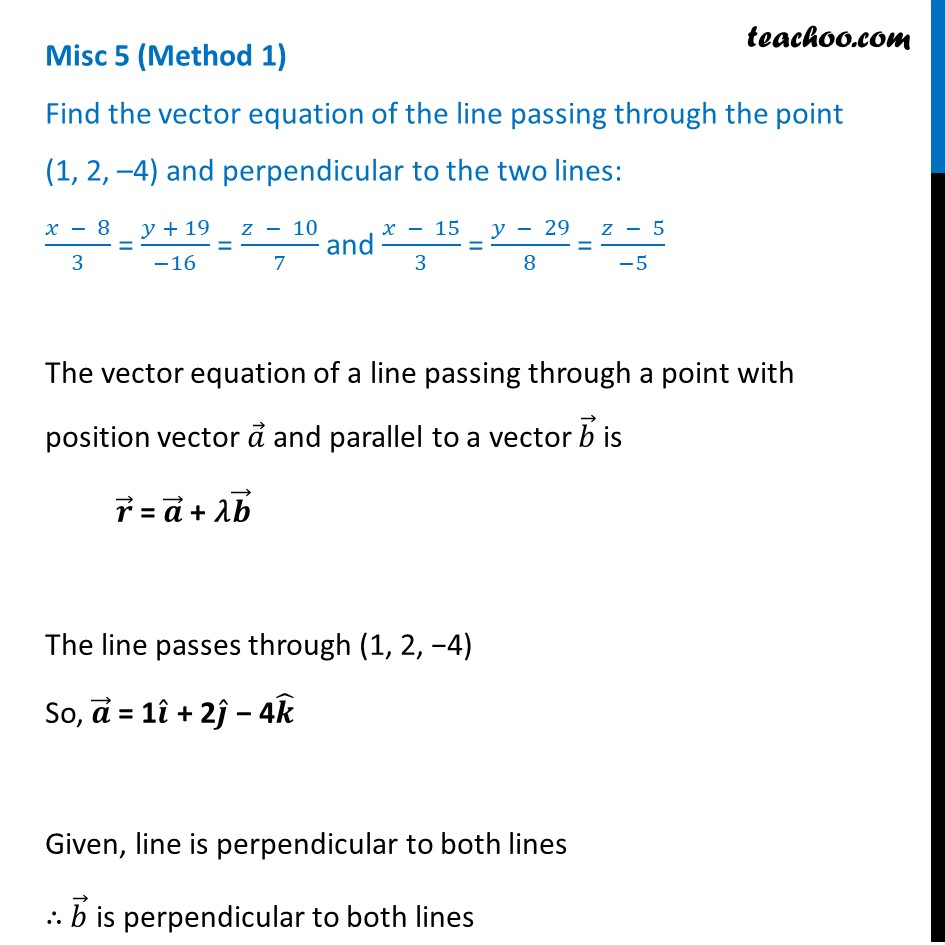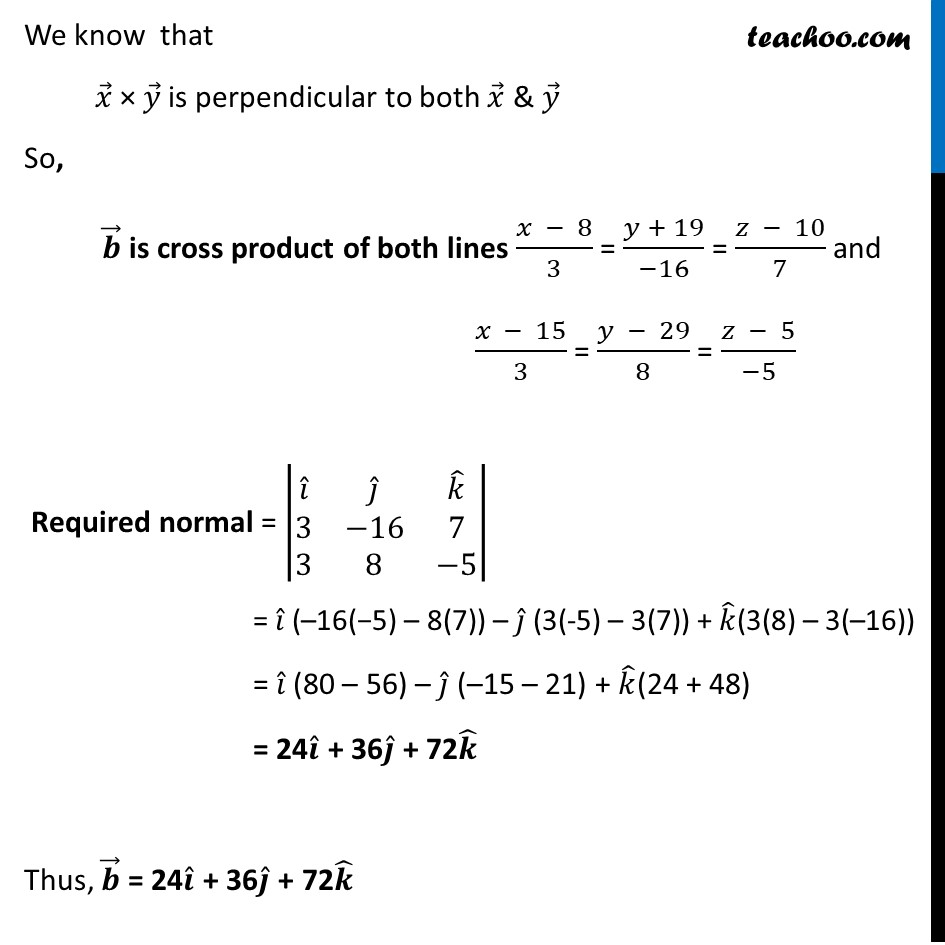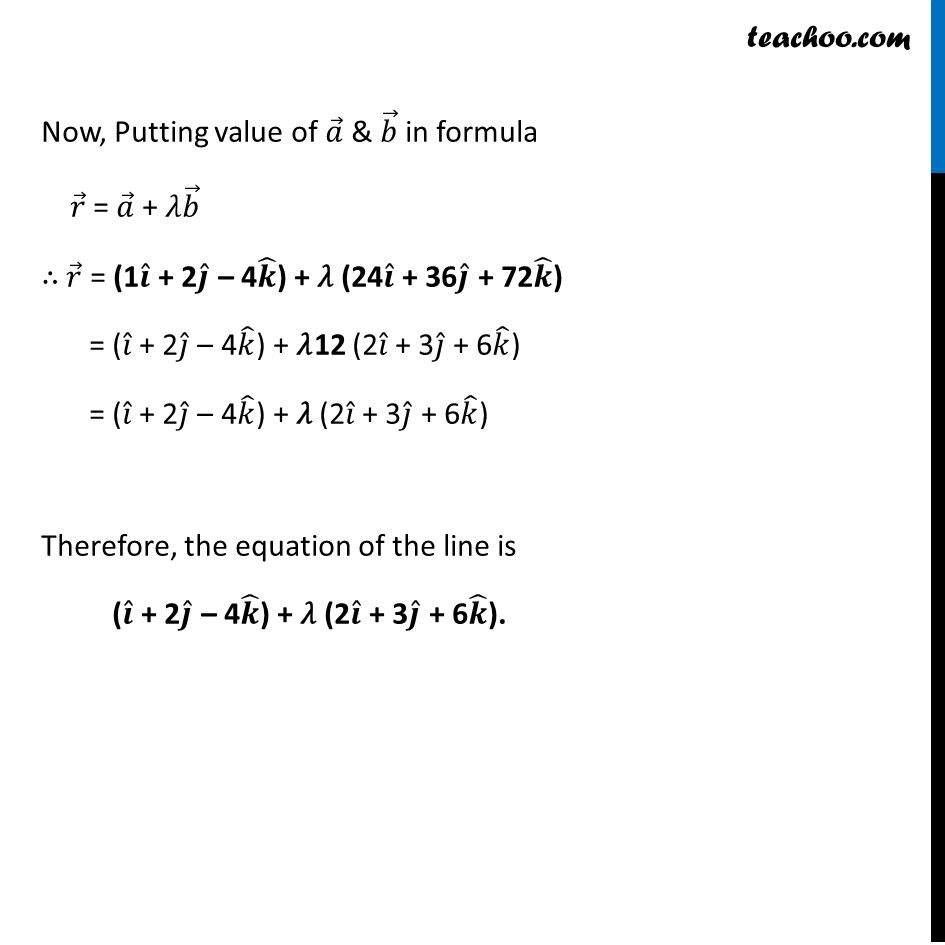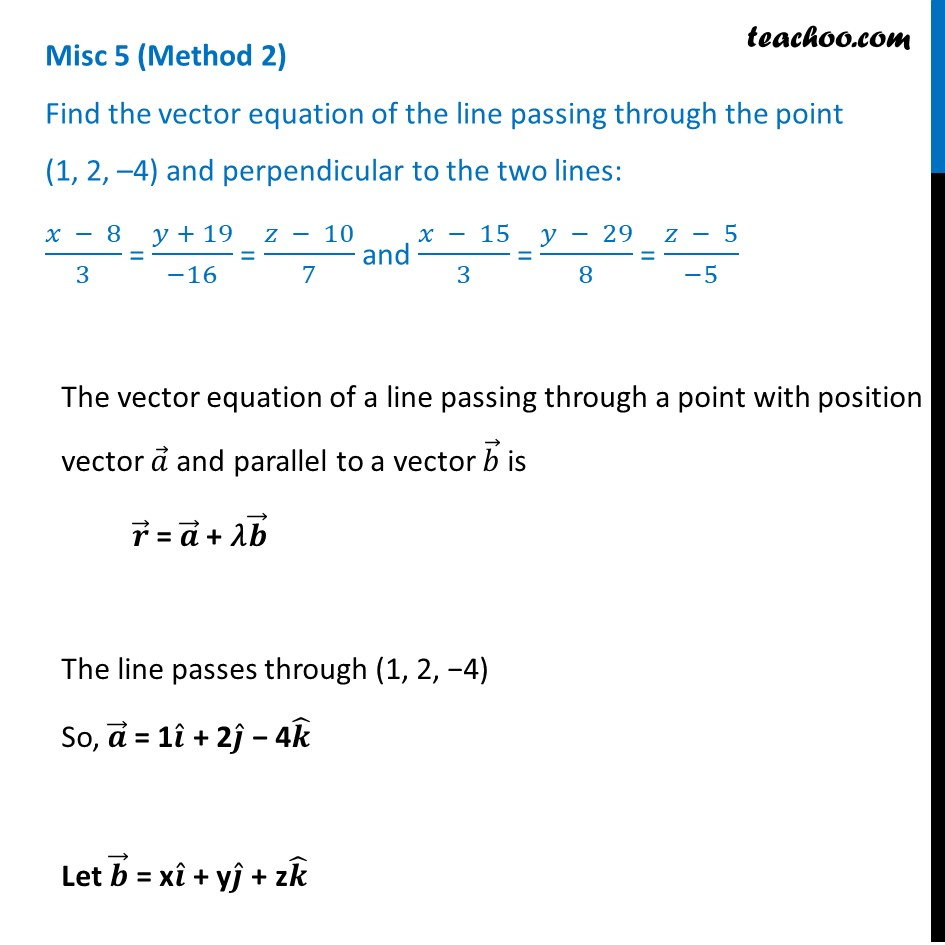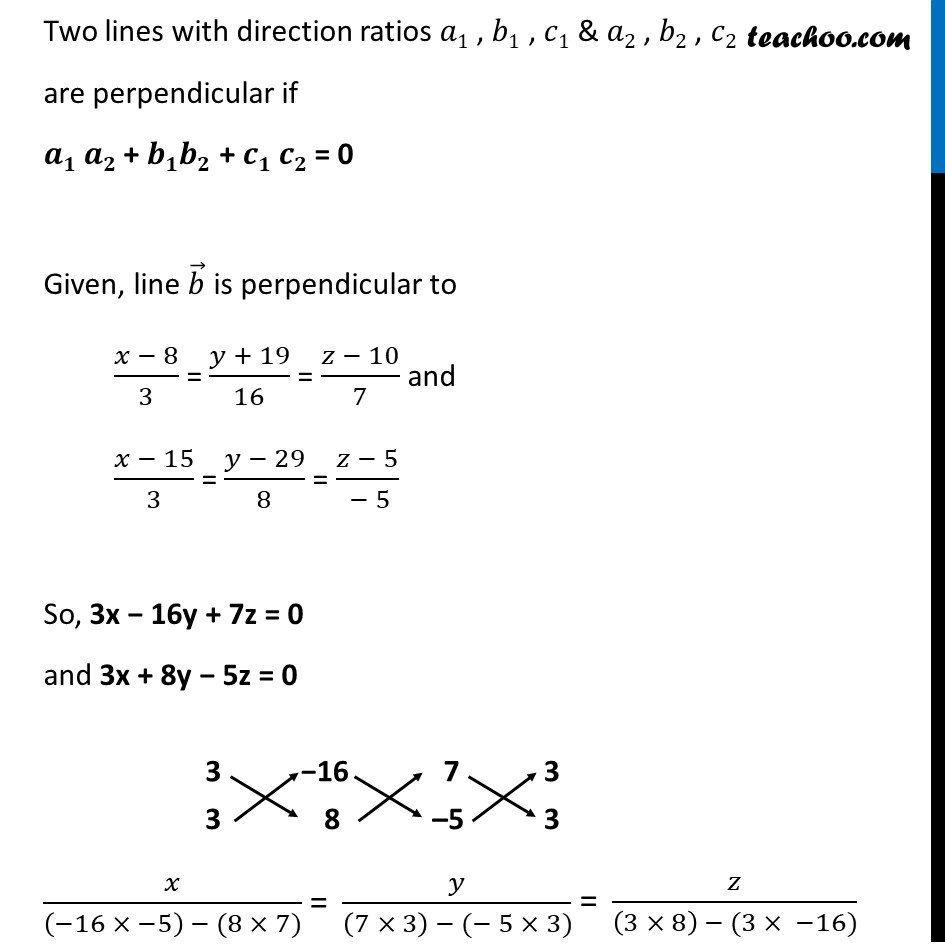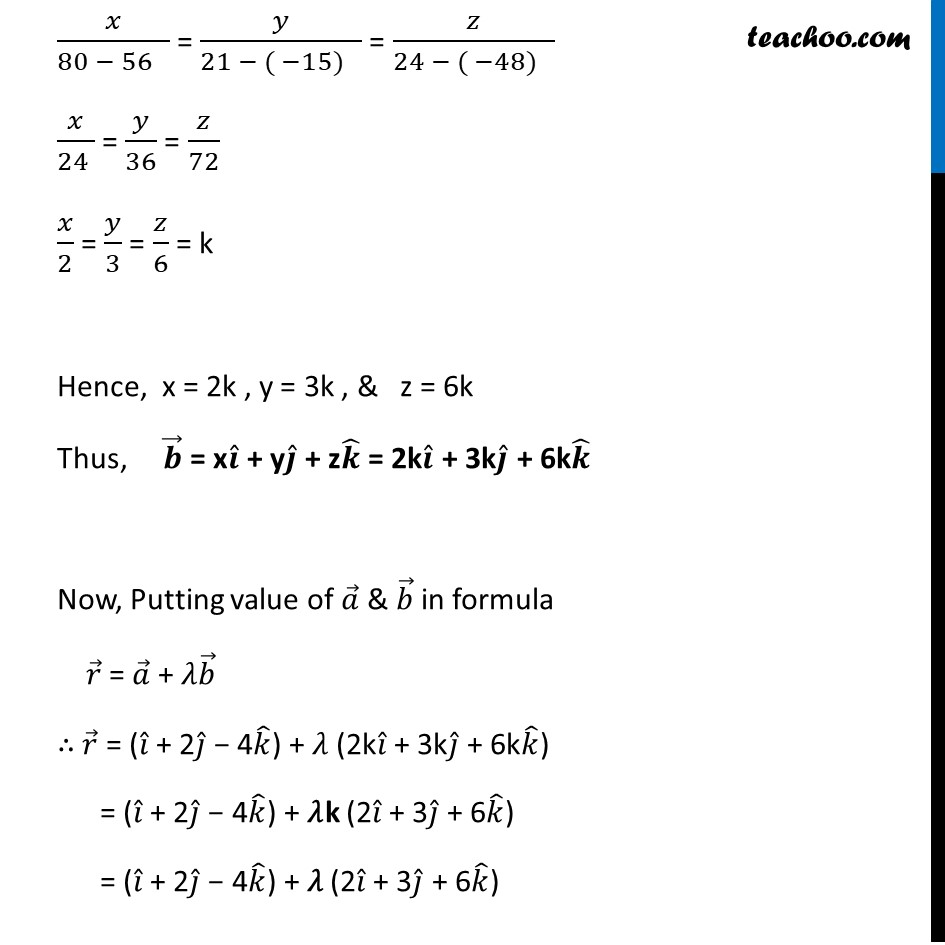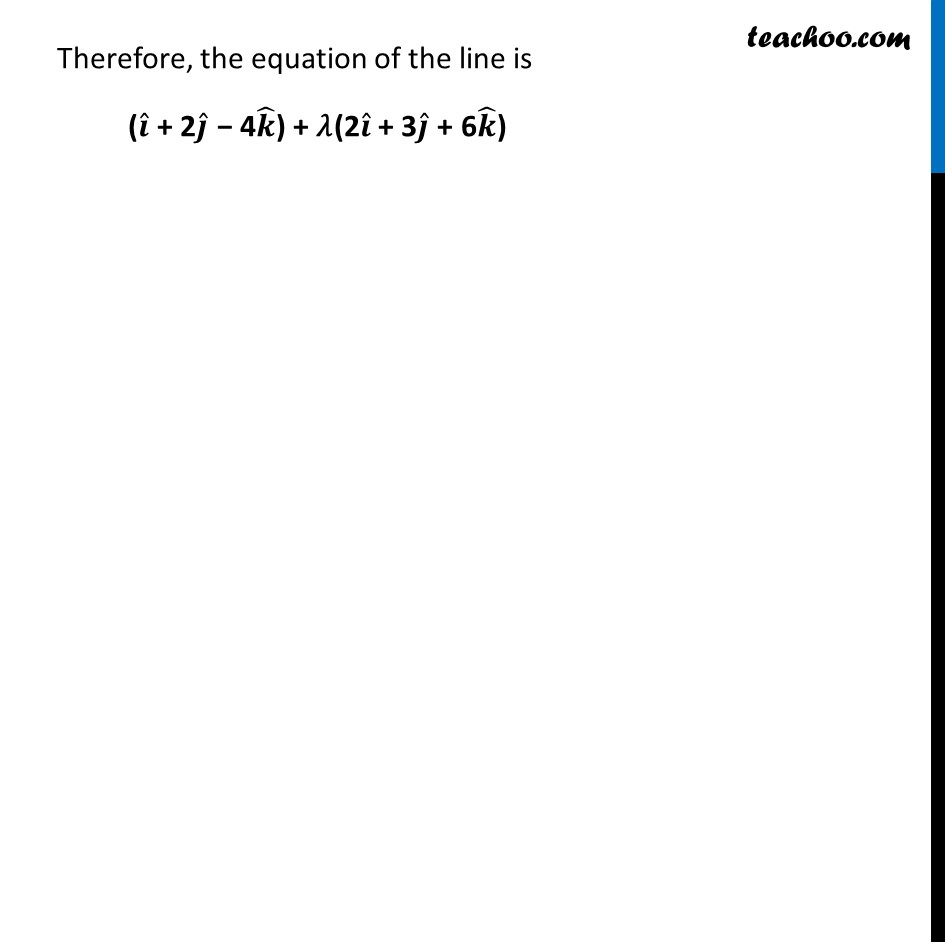Learn in your speed, with individual attention - Teachoo Maths 1-on-1 Class

### Transcript

Misc 5 (Method 1) Find the vector equation of the line passing through the point (1, 2, –4) and perpendicular to the two lines: (𝑥 − 8)/3 = (𝑦 + 19)/(−16) = (𝑧 − 10)/7 and (𝑥 − 15)/3 = (𝑦 − 29)/8 = (𝑧 − 5)/(−5) The vector equation of a line passing through a point with position vector 𝑎 ⃗ and parallel to a vector 𝑏 ⃗ is 𝒓 ⃗ = 𝒂 ⃗ + 𝜆𝒃 ⃗ The line passes through (1, 2, −4) So, 𝒂 ⃗ = 1𝒊 ̂ + 2𝒋 ̂ − 4𝒌 ̂ Given, line is perpendicular to both lines ∴ 𝑏 ⃗ is perpendicular to both lines We know that 𝑥 ⃗ × 𝑦 ⃗ is perpendicular to both 𝑥 ⃗ & 𝑦 ⃗ So, 𝒃 ⃗ is cross product of both lines (𝑥 − 8)/3 = (𝑦 + 19)/(−16) = (𝑧 − 10)/7 and (𝑥 − 15)/3 = (𝑦 − 29)/8 = (𝑧 − 5)/(−5) Required normal = |■8(𝑖 ̂&𝑗 ̂&𝑘 ̂@3&−16&7@3&8&−5)| = 𝑖 ̂ (–16(−5) – 8(7)) – 𝑗 ̂ (3(-5) – 3(7)) + 𝑘 ̂(3(8) – 3(–16)) = 𝑖 ̂ (80 – 56) – 𝑗 ̂ (–15 – 21) + 𝑘 ̂(24 + 48) = 24𝒊 ̂ + 36𝒋 ̂ + 72𝒌 ̂ Thus, 𝒃 ⃗ = 24𝒊 ̂ + 36𝒋 ̂ + 72𝒌 ̂ Now, Putting value of 𝑎 ⃗ & 𝑏 ⃗ in formula 𝑟 ⃗ = 𝑎 ⃗ + 𝜆𝑏 ⃗ ∴ 𝑟 ⃗ = (1𝒊 ̂ + 2𝒋 ̂ – 4𝒌 ̂) + 𝜆 (24𝒊 ̂ + 36𝒋 ̂ + 72𝒌 ̂) = (𝑖 ̂ + 2𝑗 ̂ – 4𝑘 ̂) + 𝜆12 (2𝑖 ̂ + 3𝑗 ̂ + 6𝑘 ̂) = (𝑖 ̂ + 2𝑗 ̂ – 4𝑘 ̂) + 𝜆 (2𝑖 ̂ + 3𝑗 ̂ + 6𝑘 ̂) Therefore, the equation of the line is (𝒊 ̂ + 2𝒋 ̂ – 4𝒌 ̂) + 𝜆 (2𝒊 ̂ + 3𝒋 ̂ + 6𝒌 ̂). Misc 5 (Method 2) Find the vector equation of the line passing through the point (1, 2, –4) and perpendicular to the two lines: (𝑥 − 8)/3 = (𝑦 + 19)/(−16) = (𝑧 − 10)/7 and (𝑥 − 15)/3 = (𝑦 − 29)/8 = (𝑧 − 5)/(−5) The vector equation of a line passing through a point with position vector 𝑎 ⃗ and parallel to a vector 𝑏 ⃗ is 𝒓 ⃗ = 𝒂 ⃗ + 𝜆𝒃 ⃗ The line passes through (1, 2, −4) So, 𝒂 ⃗ = 1𝒊 ̂ + 2𝒋 ̂ − 4𝒌 ̂ Let 𝒃 ⃗ = x𝒊 ̂ + y𝒋 ̂ + z𝒌 ̂ Two lines with direction ratios 𝑎1 , 𝑏1 , 𝑐1 & 𝑎2 , 𝑏2 , 𝑐2 are perpendicular if 𝒂𝟏 𝒂𝟐 + 𝒃𝟏𝒃𝟐 + 𝒄𝟏 𝒄𝟐 = 0 Given, line 𝑏 ⃗ is perpendicular to (𝑥 − 8)/3 = (𝑦 + 19)/16 = (𝑧 − 10)/7 and (𝑥 − 15)/3 = (𝑦 − 29)/8 = (𝑧 − 5)/( − 5) So, 3x − 16y + 7z = 0 and 3x + 8y − 5z = 0 𝑥/(80 − 56 ) = 𝑦/(21 − ( −15) ) = 𝑧/(24 − ( −48) ) 𝑥/(24 ) = 𝑦/36 = 𝑧/72 𝑥/2 = 𝑦/3 = 𝑧/6 = k Hence, x = 2k , y = 3k , & z = 6k Thus, 𝒃 ⃗ = x𝒊 ̂ + y𝒋 ̂ + z𝒌 ̂ = 2k𝒊 ̂ + 3k𝒋 ̂ + 6k𝒌 ̂ Now, Putting value of 𝑎 ⃗ & 𝑏 ⃗ in formula 𝑟 ⃗ = 𝑎 ⃗ + 𝜆𝑏 ⃗ ∴ 𝑟 ⃗ = (𝑖 ̂ + 2𝑗 ̂ − 4𝑘 ̂) + 𝜆 (2k𝑖 ̂ + 3k𝑗 ̂ + 6k𝑘 ̂) = (𝑖 ̂ + 2𝑗 ̂ − 4𝑘 ̂) + 𝜆k (2𝑖 ̂ + 3𝑗 ̂ + 6𝑘 ̂) = (𝑖 ̂ + 2𝑗 ̂ − 4𝑘 ̂) + 𝜆 (2𝑖 ̂ + 3𝑗 ̂ + 6𝑘 ̂) Therefore, the equation of the line is (𝒊 ̂ + 2𝒋 ̂ − 4𝒌 ̂) + 𝜆(2𝒊 ̂ + 3𝒋 ̂ + 6𝒌 ̂)